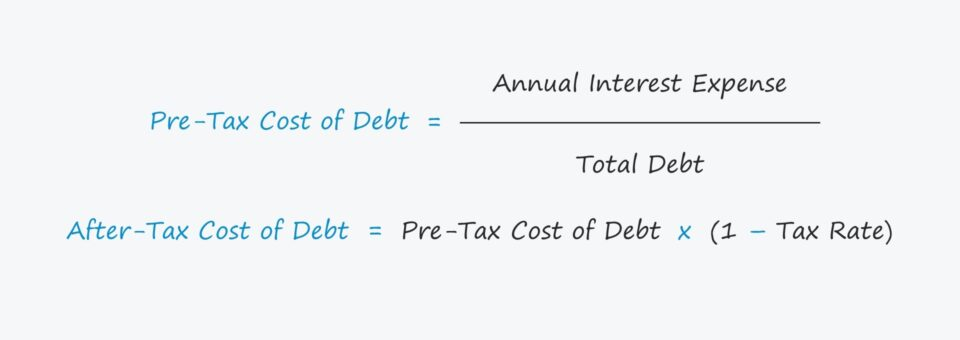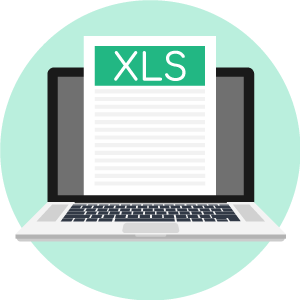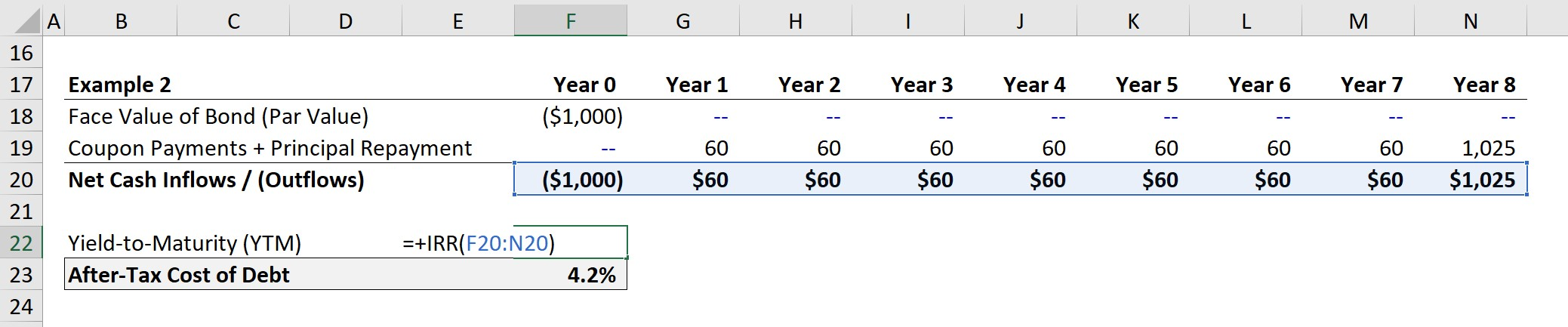# Cost of Debt (kd)

Understand the Cost of Debt Concept• From the perspective of lenders, what is the meaning behind the cost of debt concept?
• For companies with publicly-traded debt, how can the cost of debt be calculated?
• If a company is private with no public debt, can the company’s cost of debt still be estimated?
• In the calculation of WACC, why is the pre-tax cost of debt required to be “tax-affected”?

## Cost of Debt Definition

The cost of debt is the effective interest rate that a company is required to pay on its long-term debt obligations, while also being the minimum required yield expected by lenders to compensate for the potential loss of capital when lending to a borrower.

For example, a bank might lend \$1 million in debt capital to a company at an annual interest rate of 6.0% with a ten-year term.

The question here is, “Would it correct to use the 6.0% annual interest rate as the company’s cost of debt?” — to which the answer is a “No”.

On the date that the original lending terms were agreed upon, the pricing on the debt — i.e. the annual interest rate — was a contractual agreement negotiated in the past.

If the company were to attempt to raise debt in the credit markets right now, the pricing on the debt would most likely differ.

The diligence conducted by the lender used the most recent financial performance and credit metrics of the borrower as of that specific period (i.e. the past), as opposed to the current date.

• Higher Cost of Debt: If the credit health of the borrower declined since the initial date of financing, the cost of debt and risk of lending to this particular borrower increases.
• Lower Cost of Debt: In contrast, the fundamentals of the company might have improved over time (e.g. profit margin expansion, more free cash flows), which leads to a lower cost of capital and more favorable lending terms.
###### Nominal vs Effective Cost of Debt

Remember the discounted cash flow (DCF) method of valuing companies is on a “forward-looking” basis and the estimated value is a function of discounting future free cash flows (FCFs) to the present day.

With that said, the cost of debt must reflect the “current” cost of borrowing, which is a function of the company’s credit profile right now (e.g. credit ratios, scores from credit agencies).

## Pre-Tax Cost of Debt Formula

The process of estimating the cost of debt requires finding the yield on the existing debt obligations of the borrower, which accounts for two factors:

1. Nominal Interest Rate
2. Bond Market Price

The cost of debt is the interest rate that a company is required to pay in order to raise debt capital, which can be derived by finding the yield-to-maturity (YTM).

The YTM refers to the internal rate of return (IRR) of a bond, which is a more accurate approximation of the current, updated interest rate if the company tried to raise debt as of today.

Hence, the cost of debt is NOT the nominal interest rate, but rather the yield on the company’s long-term debt instruments. The nominal interest rate on debt is a historical figure, whereas the yield can be calculated on a current basis.

While using the market-based yield from sources like Bloomberg is certainly the preferred option, the pre-tax cost of debt can be manually calculated by dividing the annual interest expense by the total debt obligation — otherwise known as the “effective interest rate”.

###### Pre−Tax Cost of Debt Formula
• Pre-Tax Cost of Debt = Annual Interest Expense / Total Debt

The effective interest rate is defined as the blended average interest rate paid by a company on all its debt obligations, denoted in the form of a percentage.

###### Bond Equivalent Yield (BEY) vs Effective Annual Yield (EAY)

On the Bloomberg terminal, the quoted yield refers to a variation of yield-to-maturity (YTM) called the “bond equivalent yield” (or BEY).

The “effective annual yield” (EAY) could also be used (and could be argued to be more accurate), but the difference tends to be marginal and is very unlikely to have a material impact on the analysis.

The EAY is the annualized yield that incorporates compounding, whereas BEY annualizes a bond’s semi-annual yield by simply doubling it (e.g. 3.0% x 2 = 6%) — which while a frequently criticized convention, is still widely used in practice.

## Cost of Debt — Public vs Private Companies

Calculating the cost of debt differs depending on whether the company is publicly traded or private:

• Publicly-Traded Companies: The cost of debt should reflect the yield to maturity (YTM) on the company’s long-term debt.
• Privately-Held Companies: If the company is private and the yield cannot be found on sources like Bloomberg, the cost of debt can be estimated from the yield on debt of comparable companies of equivalent risk.
###### “Synthetic” Credit Ratings

For companies with no publicly-traded debt, the options to estimate the cost of debt are as follows:

• No Public Debt: If the company does not have any debt trading in the credit markets, the default spread associated with the comparable credit rating (i.e. S&P, Moody’s) could be added to the risk-free rate.
• Private Companies: While not ideal, the interest coverage ratio (EBIT/Interest Expense) could be calculated and then matched to the default spread for a so-called “synthetic” credit rating — which is published and frequently updated by NYU Professor Damodaran.

## After-Tax Cost of Debt Formula

In the calculation of the weighted average cost of capital (WACC), the formula uses the “after-tax” cost of debt.

The reason why the pre-tax cost of debt must be tax-affected is due to the fact that interest is tax-deductible, which effectively creates a “tax shield” — i.e. the interest expense reduces the taxable income (earnings before taxes, or EBT) of a company.

###### After−Tax Cost of Debt Formula
• After−Tax Cost of Debt = Pre-Tax Cost of Debt x (1 – Tax Rate)

Note the tax benefits of debt financing are accounted for in the company’s discount rate inclusive of all capital providers (or the WACC), which is why the DCF uses the net operating profits after tax (NOPAT) in its calculation to avoid double-counting.

The difference between the pre-tax cost of debt and the after-tax cost of debt is attributable to how interest expense reduces the amount of taxes paid, unlike dividends issued to common or preferred equity holders.

## Cost of Debt Calculator – Excel Template

We’ll now move to a modeling exercise, which you can access by filling out the form below.Submitting ...

## Cost of Debt Model Assumptions

As a preface for our modeling exercise, we’ll be calculating the cost of debt in Excel using two distinct approaches, but with identical model assumptions.

###### Model Assumptions
• Face Value of Bond (Par Value): \$1,000
• Current Market Price of Bond: \$1,025
• Annual Coupon Rate (%): 6.0%
• Term (# of Years): 8 Years

## Cost of Debt Calculation [Example 1]

Provided with these figures, we can calculate the interest expense by dividing the annual coupon rate by two (to convert to a semi-annual rate) and then multiplying by the face value of the bond.

• Semi-Annual Interest Expense = (6.0% / 2) * \$1,000 = \$30Each year, the lender will receive \$30 in total interest expense twice.

Next, we’ll calculate the interest rate using a slightly more complex formula in Excel.

###### Interest Rate “RATE” Excel Function
• Semi-Annual Interest Rate (%) =RATE(Term * 2, Semi-Annual Interest Expense, Semi-Annual Interest Rate, – Current Price of Bond, Face Value of Bond)
• Semi-Annual Interest Rate (%) = 2.8%Since the interest rate is a semi-annual figure, we must convert it to an annualized figure by multiplying it by two.

• Pre-Tax Cost of Debt = \$2.8% x 2 = 5.6%To arrive at the after-tax cost of debt, we multiply the pre-tax cost of debt by (1 — tax rate).

• After-Tax Cost of Debt = 5.6% x (1 – 25%) = 4.2%## Cost of Debt Calculation [Example 2]

For the next section of our modeling exercise, we’ll calculate the cost of debt but in a more visually illustrative format.

In our table, we have listed the two cash inflows and outflows from the perspective of the lender, since we’re calculating the YTM from their viewpoint.

1. Cash Outflow (-): Face Value of Bond
2. Cash Inflow (+): Coupon Payments + Principal Repayment

The face value of the bond is \$1,000, which is linked with a negative sign placed in front to indicate it is a cash outflow.

In the subsequent steps, we will enter the annual coupon payments across the lending term.

• Coupon Payments = \$30 x 2 = \$60

The current market price of the bond, \$1,025, is then input into the Year 8 cell.

Using the “IRR” function in Excel, we can calculate the yield-to-maturity (YTM) as 5.6%, which is equivalent to the pre-tax cost of debt.Therefore, the final step is to tax-affect the YTM, which comes out to an estimated 4.2% cost of debt once again, as shown by our completed model output.Step-by-Step Online Course

#### Everything You Need To Master Financial Modeling

Enroll in The Premium Package: Learn Financial Statement Modeling, DCF, M&A, LBO and Comps. The same training program used at top investment banks.Inline FeedbacksLearn Financial Modeling Online

Everything you need to master financial and valuation modeling: 3-Statement Modeling, DCF, Comps, M&A and LBO.

X

The Wall Street Prep Quicklesson Series

7 Free Financial Modeling Lessons

Get instant access to video lessons taught by experienced investment bankers. Learn financial statement modeling, DCF, M&A, LBO, Comps and Excel shortcuts.